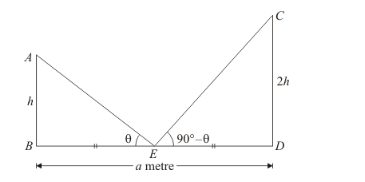# Two persons are a metres apart and the height of one is double that of the other.

Question:

Two persons are a metres apart and the height of one is double that of the other. If from the middle point of the line joining their feet, an observer finds the angular elevation of their tops to be complementary, then the height of the shorter post is

(a) $\frac{a}{4}$

(b) $\frac{a}{\sqrt{2}}$

(c) $a \sqrt{2}$

(d) $\frac{a}{2 \sqrt{2}}$

Solution:

Let AB and CD be the two persons such that AB < CD.

Then, let AB = h so that CD = 2h

Now, the given information can be represented as,Here, E is the midpoint of BD.

We have to find height of the shorter person.

So we use trigonometric ratios.

In triangle ECD,

$\tan \angle C E D=\frac{C D}{E D}$

$\Rightarrow \tan \left(90^{\circ}-\theta\right)=\frac{2 h}{\left(\frac{a}{2}\right)}$

$\Rightarrow \cot \theta=\frac{4 h}{a}$.......(1)

Again in triangle ABE,

$\Rightarrow \tan \angle A E B=\frac{A B}{B E}$

$\Rightarrow \tan \theta=\frac{h}{\left(\frac{a}{2}\right)}$

$\Rightarrow \frac{1}{\cot \theta}=\frac{2 h}{a}$

$\Rightarrow \frac{a}{4 h}=\frac{2 h}{a}$

$\Rightarrow a^{2}=8 h^{2}$

$\Rightarrow h=\frac{a}{2 \sqrt{2}}$

Hence the correct option is $d$.